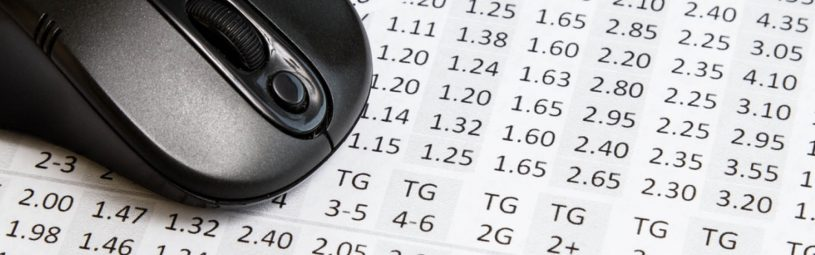How do decimal odds work in betting?

June 27th, 2017

Betting and odds go together like Friday night and a pint down the local. You just can’t have one without the other. And just like there are different things to bet on, there are different types of odds, too. You can find out more about fractional odds in this post but read on for our answer to the question “how do decimal odds work?”

How do decimal odds work in betting?

Decimal odds, also known as European odds, are popular because of their simplicity. They’re shown as two figures separated by a full stop. For example: 1.25, 2.30 or 6.4. Punters use decimal odds to work out which price is better value for their bet by multiplying their chosen stake by the decimal price (£10 x 2.30 = £23). Calculated returns include the bet stake.

What are decimal odds?

Decimal odds tell us two things:

1. Price – allowing you to calculate exactly how much money you will win if your bet wins.
2. Probability – the likelihood of your chosen bet actually winning.

But unlike fractional odds that show the potential returns without your stake, decimal odds represent the return from your bet including your stake.

Decimal ‘odds against’ prices:

• 6.0 – £1 stake x 6 = £6 returns
• 6.0 – £5 stake x 6 = £30 returns
• or 6.0 – £10 stake x 6 = £50 returns

Bets placed at ‘evens’:

• 2.0 – £1 stake x 2 = £2 returns
• 2.0 – £5 stake x 2 = £10 returns
• or 2.0 – £10 stake x 2 = £20 returns

Decimal ‘odds on’ prices:

• 1.5 – £1 stake x 1.5 = £1.50 returns
• 1.5 – £5 stake x 1.5 = £7.50 returns
• or 1.5 – £10 stake x 1.5 = £15 winnings

Other types of odds

An alternative format to decimal odds are fractional odds. There’s no mathematical difference between the two types. Instead, it’s just a different way to display the same information. Some people prefer decimals while other prefer fractions.

What do odds mean?

Odds are simply a reflection of the implied chance, or likelihood, of the outcome of an event.

Let’s look at tennis, where Andy Murray is playing an early round match against a relatively unknown player. The bookmakers decide that Andy has an 80% chance of winning the match, therefore giving his opponent a 20% chance.

We’ll assume that we’re dealing with a non-profit making bookmaker. To price up their book they need to split it 80% / 20% = 100%

In decimal odds format this is very easy: they simply take 100 (the total chance of all outcomes happening in an event will always be 100%), and divide it by Andy’s 80% chance:

100% ÷ 80% = Decimal Odds of 1.25

His opponent’s odds are:

Work out a bet return using decimal odds

STAKE x DECIMAL ODDS = RETURN

If you stake £100 on Andy to win, and he does, you’ll get £125 back from the bookmaker. This includes your initial stake, so you’ll have won £25:

Probability

By using the decimal odds you can calculate the likelihood of the outcome of an event actually happening. For example:

• 6.0 is calculated as: 100 / 6.0 = 0.16 or 16% probability
• 2.0 is calculated as: 100 / 2.0 = 0.50 or 50% probability
• 1.5 is calculated as: 100 / 1.5 = 0.66  or 66% probability

Converting decimal odds to implied probability

It’s also easier to work out the reverse of decimal odds, i.e. convert them into the implied chance of the outcome happening as you simply divide 100 by the decimal odds:

Converting fractional odds to decimal

Although it’s easy to change all your online bookmakers to display the odds as decimals, there may be times when that’s not an option. It’s useful to know how to convert fractional odds to their decimal equivalent. Fortunately, it’s very easy too: just divide the 1st number by the second and add 1.

So taking Andy’s odds above their fractional equivalent would be 1 / 4

Converting decimal odds to fractional

It’s really straightforward. Just subtract 1.00 from the decimal price, convert the price to a fraction and then simplify that fraction are far as possible. For example:

• 6.0 as fractional odds would be 5/1
(6.0 – 1 = 5) = 5/1
• 8.5 as fractional odds would be 15/2
(8.5 – 1 = 7.5)  = 15/2
• 1.5 as decimal odds would be 1/2
(1.5 – 1 = 0.5) = 1/2

How to use decimal odds to make money

Matched betting is a clever way to turn bookmakers’ free bets and promotions into real money. It involves odds and placing bets, but it’s not gambling. The process of matched betting means that you eliminate the risks usually associated with gambling. And you don’t have to do it on you’re own. You don’t even need to know anything about sport!

Here at OddsMonkey, we have a range of software, services and support matched betting tool called the OddsMatcher. Using decimal odds, it finds the best matches (between back and lay odds) and helps you extract real value. Oddsmatcher is the main tool in any matched bettor’s arsenal and does all the hard work is done for you! It’s one of the best ways to help you release those free bets and bonuses and make some extra cash.

Why use decimal odds in matched betting?

• It’s much easier to compare the closeness in the odds for matching those bets.
• The higher the odds, the less the chance of the event occurring.
• The lower the odds, the higher the chance of the event occurring.

So, to wrap up, decimal odds are an easy way to compare the odds at both the bookmaker and the exchange to find the best matched bets.

Download our free introduction to matched betting to find out more: